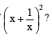Courses

# Algebraic Expressions And Identities -MCQ

## 10 Questions MCQ Test Class 8 Mathematics by VP Classes | Algebraic Expressions And Identities -MCQ

Description
This mock test of Algebraic Expressions And Identities -MCQ for Class 8 helps you for every Class 8 entrance exam. This contains 10 Multiple Choice Questions for Class 8 Algebraic Expressions And Identities -MCQ (mcq) to study with solutions a complete question bank. The solved questions answers in this Algebraic Expressions And Identities -MCQ quiz give you a good mix of easy questions and tough questions. Class 8 students definitely take this Algebraic Expressions And Identities -MCQ exercise for a better result in the exam. You can find other Algebraic Expressions And Identities -MCQ extra questions, long questions & short questions for Class 8 on EduRev as well by searching above.
QUESTION: 1

Solution:
QUESTION: 2

Solution:
QUESTION: 3

### What kind of polynomial is p q r?

Solution: P,q,r are not terms they are just number hence it is a monomial which is known as single term.
QUESTION: 4

The value of x2 – 5 at x = –1 is:

Solution:
QUESTION: 5

a2 – b2 is the product of:

Solution:
QUESTION: 6

Which of the following in the value ofSolution:
QUESTION: 7

Which of the following is obtained by subtracting x2 – y2 from y2 – x2?

Solution:
QUESTION: 8

Which of the following is the degree of x3 – x2y2 – 8y2 + 2?

Solution:
QUESTION: 9

Which of the following is the value of 5x25 – 3x32 + 2x –12 at x = 1?

Solution:
QUESTION: 10

What is the product of (x + a) and (x + b)?

Solution: